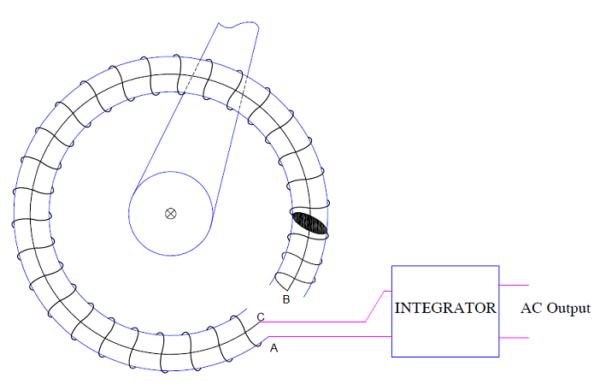# Concept of Rogowski Coil and its Working

### What is Rogowski Coil?

Rogowski Coil is an electrical device used for the measurement of alternating (AC) current. It can measure high speed transient current, pulsed current as well as power frequency AC current. It is simply a toroidal coil named after German physicist Walter Rogowski.

A Rogowski coil is evenly wound coil on a non-magnetic former. This non-megnatic former is either plastic or other flexible material. The wiring wound is made in such a way that the end point is brought to the starting point along the central axis of the former. This is shown in figure below.In the above figure, the starting point of coil is A and end point is B. The winding wire B is brought to starting point A through the central axis up to point C. Thus the two terminal A and C of the coil are at the same side. These terminals are connected to electronic Intergrator Circuit to get output voltage proportional to the current.

### Working Principle of Rogowski Coil

The working of Rogowski Coil is based on Faraday’s Law of Electromagnetic Induction. This coil surrounds the conductor whose current is to be measured. Since any current carrying conductor creates magnetic field in its surrounding, this field links with the Rogowski Coil. Due to this linkage, an emf is induced in the coil whose magnitude Is proportional to the rate of change of current in the conductor.

v = Ldi/dt where L is the inductance of coil.

Since inductance of coil is given as

L = μ0AN/l

Where A = Cross-sectional area of coil = πr2

l = 2πr = Length of coil

N = Number of turns in the coil

μ0 = Magnetic permeability of air

= 2πx10-7 A-m

To obtain output voltage proportional to the conductor current, an electronic Integrator Circuit is connected across the Rogowski coil terminals. Therefore the output voltage is given as

V0 = ∫Ldi/dt

= (μ0AN/l)I + C

where C is constant of integration.

Usually this constant of integration is made zero by design, therefore

V0 = (μ0AN/l)I